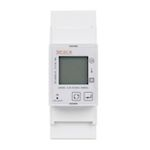# # TuYa TS0601_3_phase_clamp_meter_relay

ModelTS0601_3_phase_clamp_meter_relay
VendorTuYa
Description3-phase clamp power meter with relay
Exposesswitch (state), power, energy, produced_energy, voltage_a, voltage_b, voltage_c, power_a, power_b, power_c, current_a, current_b, current_c, linkquality
PictureWhite-labelWenzhou Taiye Electric TAC7361C BI

## # Options

How to use device type specific configuration

• `energy_precision`: Number of digits after decimal point for energy, takes into effect on next report of device. The value must be a number with a minimum value of `0` and with a with a maximum value of `3`

• `energy_calibration`: Calibrates the energy value (percentual offset), takes into effect on next report of device. The value must be a number.

• `power_precision`: Number of digits after decimal point for power, takes into effect on next report of device. The value must be a number with a minimum value of `0` and with a with a maximum value of `3`

• `power_calibration`: Calibrates the power value (percentual offset), takes into effect on next report of device. The value must be a number.

## # Exposes

### # Switch

The current state of this switch is in the published state under the `state` property (value is `ON` or `OFF`). To control this switch publish a message to topic `zigbee2mqtt/FRIENDLY_NAME/set` with payload `{"state": "ON"}`, `{"state": "OFF"}` or `{"state": "TOGGLE"}`. It's not possible to read (`/get`) this value.

### # Power (numeric)

Instantaneous measured power. Value can be found in the published state on the `power` property. It's not possible to read (`/get`) or write (`/set`) this value. The unit of this value is `W`.

### # Energy (numeric)

Sum of consumed energy. Value can be found in the published state on the `energy` property. It's not possible to read (`/get`) or write (`/set`) this value. The unit of this value is `kWh`.

### # Produced_energy (numeric)

Sum of produced energy. Value can be found in the published state on the `produced_energy` property. It's not possible to read (`/get`) or write (`/set`) this value. The unit of this value is `kWh`.

### # Voltage_a (numeric)

Measured electrical potential value (phase A). Value can be found in the published state on the `voltage_a` property. It's not possible to read (`/get`) or write (`/set`) this value. The unit of this value is `V`.

### # Voltage_b (numeric)

Measured electrical potential value (phase B). Value can be found in the published state on the `voltage_b` property. It's not possible to read (`/get`) or write (`/set`) this value. The unit of this value is `V`.

### # Voltage_c (numeric)

Measured electrical potential value (phase C). Value can be found in the published state on the `voltage_c` property. It's not possible to read (`/get`) or write (`/set`) this value. The unit of this value is `V`.

### # Power_a (numeric)

Instantaneous measured power (phase A). Value can be found in the published state on the `power_a` property. It's not possible to read (`/get`) or write (`/set`) this value. The unit of this value is `W`.

### # Power_b (numeric)

Instantaneous measured power (phase B). Value can be found in the published state on the `power_b` property. It's not possible to read (`/get`) or write (`/set`) this value. The unit of this value is `W`.

### # Power_c (numeric)

Instantaneous measured power (phase C). Value can be found in the published state on the `power_c` property. It's not possible to read (`/get`) or write (`/set`) this value. The unit of this value is `W`.

### # Current_a (numeric)

Instantaneous measured electrical current (phase A). Value can be found in the published state on the `current_a` property. It's not possible to read (`/get`) or write (`/set`) this value. The unit of this value is `A`.

### # Current_b (numeric)

Instantaneous measured electrical current (phase B). Value can be found in the published state on the `current_b` property. It's not possible to read (`/get`) or write (`/set`) this value. The unit of this value is `A`.

### # Current_c (numeric)

Instantaneous measured electrical current (phase C). Value can be found in the published state on the `current_c` property. It's not possible to read (`/get`) or write (`/set`) this value. The unit of this value is `A`.

Link quality (signal strength). Value can be found in the published state on the `linkquality` property. It's not possible to read (`/get`) or write (`/set`) this value. The minimal value is `0` and the maximum value is `255`. The unit of this value is `lqi`.